Scan QR code or get instant email to install app

Question:

# 1212Which of these is equivalent to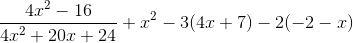?

A 1212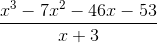explanation

1212Factor the numerator and denominator of the fraction: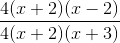; notice that 4(x+2) can be eliminated.
Distribute the remaining terms in the expression: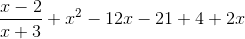Combine like terms to simplify: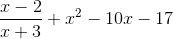Multiply the non fraction terms by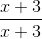to find a common denominator and combine each term: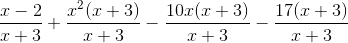Distribute through the numerator and place over the common denominator: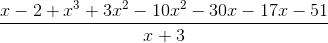Combine like terms: